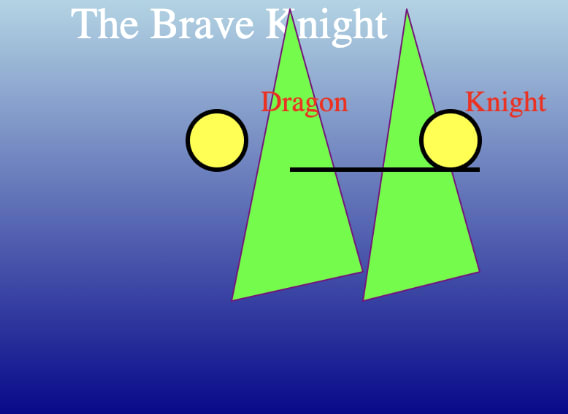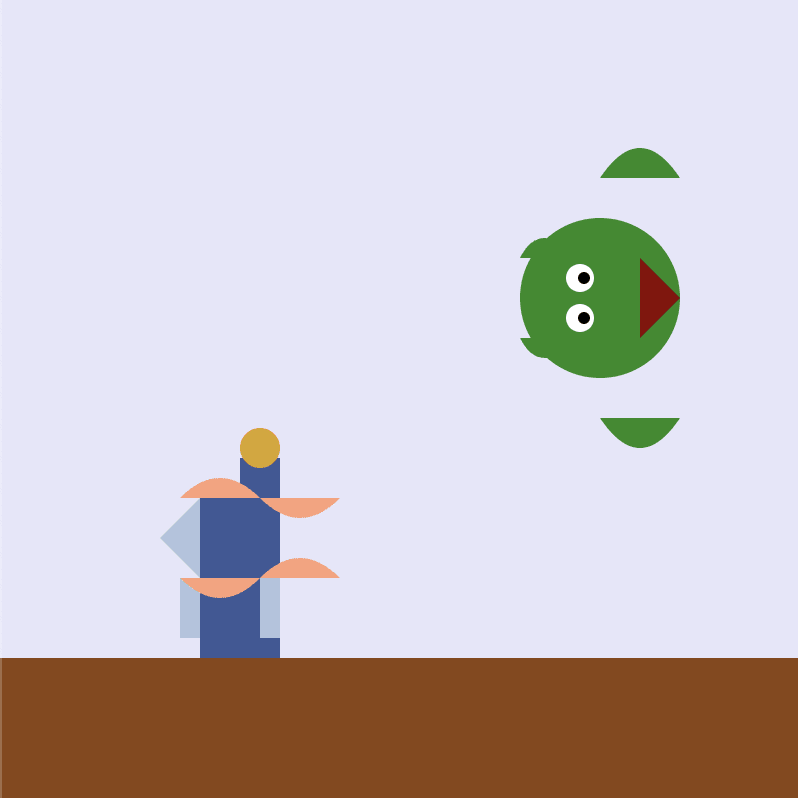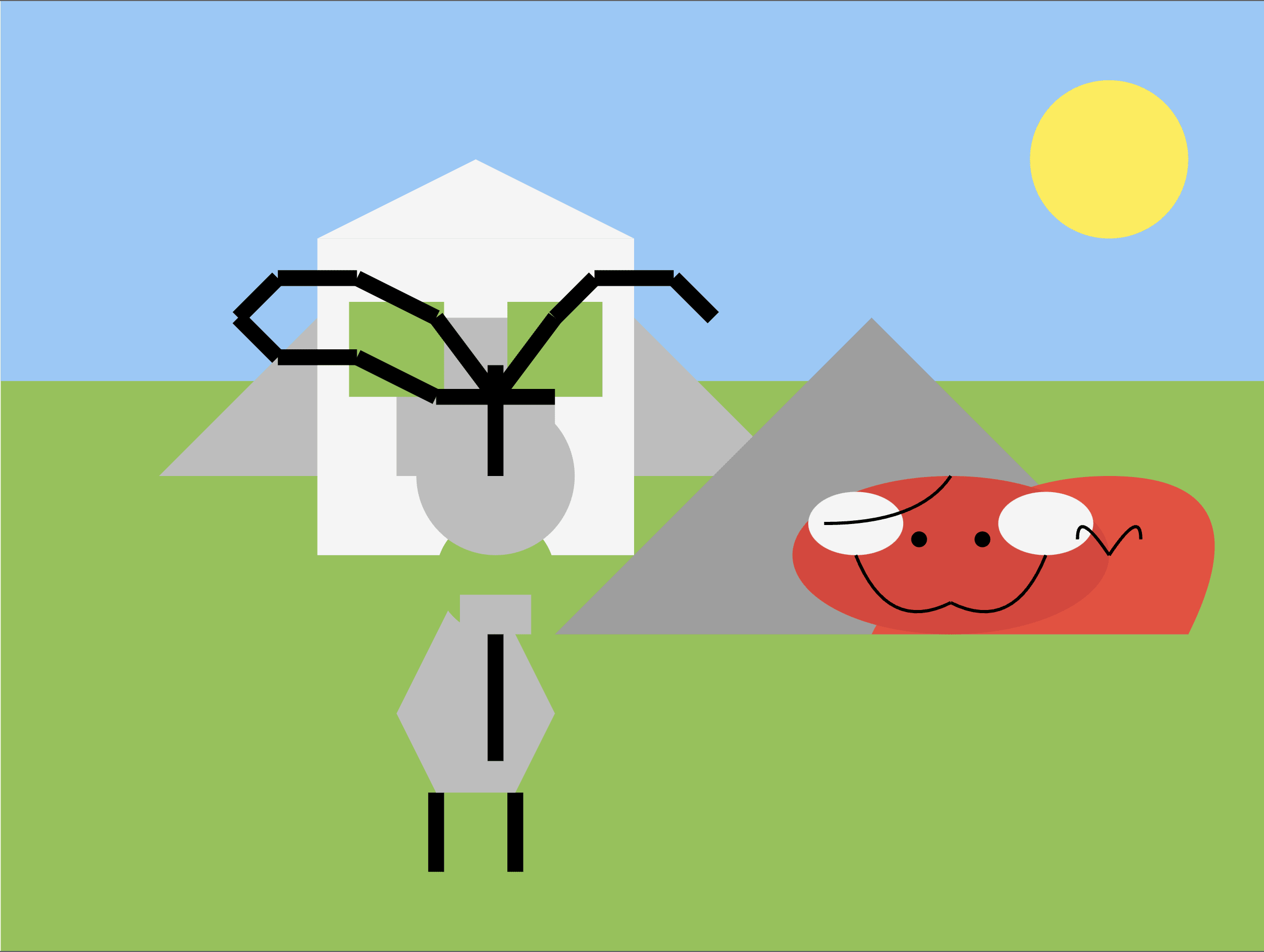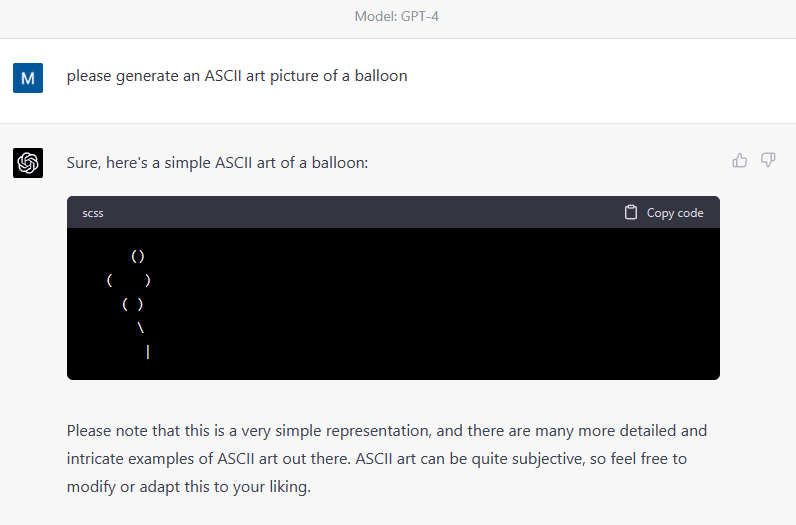# GPT-4 and ASCII Images?

post by carterallen · 2023-03-19T15:46:58.888Z · LW · GW · 17 comments

This is a question post.

Does anyone know whether GPT-4 successfully generates ASCII art?

GPT-3.5 couldn't:

Which makes sense, cause of the whole words-can't-convey-phenomena thing.

I'd expect multimodality to solve this problem, though?

answer by jrh206 · 2023-03-19T17:08:10.843Z · LW(p) · GW(p)
answer by Raemon · 2023-03-20T01:24:44.669Z · LW(p) · GW(p)

Note that ASCII art isn't the only kind of art. I just asked GPT4 and Claude to both make SVGs of a knight fighting a dragon.

Here's Claude's attempt:And GPT4s:

I asked them both to make it more realistic. Claude responded with the exact same thing with some extra text, GPT4 returned:

I asked followed up asking it for more muted colors and a simple background, and it returned:comment by Raemon · 2023-03-20T01:46:40.750Z · LW(p) · GW(p)

I tried again, accidentally using GPT3.5 this time, which initially gave something really lame, but then said "more realistic please", and it gave me:comment by Raemon · 2023-03-20T03:47:09.989Z · LW(p) · GW(p)

It responded with this image:

code:

``````<svg width="300" height="300" viewBox="0 0 300 300" xmlns="http://www.w3.org/2000/svg">
<!-- Background circle -->
<circle cx="150" cy="150" r="140" fill="none" stroke="black" stroke-width="2"/>

<!-- Body -->
<ellipse cx="150" cy="100" rx="30" ry="40" fill="none" stroke="black" stroke-width="2"/>
<rect x="140" y="140" width="20" height="60" fill="none" stroke="black" stroke-width="2"/>
<line x1="100" y1="140" x2="200" y2="140" stroke="black" stroke-width="2"/>

<!-- Legs -->
<line x1="150" y1="200" x2="110" y2="270" stroke="black" stroke-width="2"/>
<line x1="150" y1="200" x2="190" y2="270" stroke="black" stroke-width="2"/>
<line x1="150" y1="200" x2="90" y2="270" stroke="black" stroke-width="2"/>
<line x1="150" y1="200" x2="210" y2="270" stroke="black" stroke-width="2"/>

<!-- Arms -->
<line x1="140" y1="140" x2="70" y2="140" stroke="black" stroke-width="2"/>
<line x1="160" y1="140" x2="230" y2="140" stroke="black" stroke-width="2"/>
<line x1="140" y1="140" x2="60" y2="80" stroke="black" stroke-width="2"/>
<line x1="160" y1="140" x2="240" y2="80" stroke="black" stroke-width="2"/>

<circle cx="150" cy="100" r="20" fill="none" stroke="black" stroke-width="2"/>
</svg>``````

Then I asked if it could do the Vitruvian Man as Ascii art, and it said:

``````         O
-|-|-
|
/|\
/ \``````
comment by cwillu (carey-underwood) · 2023-03-20T02:06:20.975Z · LW(p) · GW(p)

Any chance you have the generated svg's still, not just the resulting bitmap render?

Replies from: Raemon
comment by Raemon · 2023-03-20T02:18:23.792Z · LW(p) · GW(p)

Here was the final one:

``````
<svg viewBox="0 0 800 600" xmlns="http://www.w3.org/2000/svg">
<!-- Field -->
<rect x="0" y="0" width="100%" height="100%" fill="#8BC34A"/>
<!-- Sky and sun -->
<rect x="0" y="0" width="100%" height="40%" fill="#90CAF9"/>
<circle cx="700" cy="100" r="50" fill="#FFEB3B"/>
<!-- Mountains -->
<polygon points="100,300 300,100 500,300" fill="#BDBDBD"/>
<polygon points="350,400 550,200 750,400" fill="#9E9E9E"/>
<!-- Castle -->
<rect x="200" y="150" width="200" height="200" fill="#F5F5F5"/>
<rect x="250" y="200" width="100" height="100" fill="#BDBDBD"/>
<rect x="220" y="190" width="60" height="60" fill="#8BC34A"/>
<rect x="320" y="190" width="60" height="60" fill="#8BC34A"/>
<rect x="290" y="230" width="20" height="60" fill="#BDBDBD"/>
<polygon points="200,150 400,150 300,100" fill="#F5F5F5"/>
<!-- Dragon -->
<path d="M 550 400 Q 600 300 700 300 Q 800 300 750 400 Z" fill="#F44336"/>
<ellipse cx="600" cy="350" rx="100" ry="50" fill="#E53935"/>
<ellipse cx="660" cy="330" rx="30" ry="20" fill="#F5F5F5"/>
<ellipse cx="540" cy="330" rx="30" ry="20" fill="#F5F5F5"/>
<circle cx="620" cy="340" r="5" fill="#000"/>
<circle cx="580" cy="340" r="5" fill="#000"/>
<path d="M 600 380 Q 640 400 660 350" stroke="#000" stroke-width="2" fill="none"/>
<path d="M 600 380 Q 560 400 540 350" stroke="#000" stroke-width="2" fill="none"/>
<path d="M 520 330 Q 580 330 600 300" stroke="#000" stroke-width="2" fill="none"/>
<path d="M 700 350 Q 680 320 680 340" stroke="#000" stroke-width="2" fill="none"/>
<path d="M 700 350 Q 720 320 720 340" stroke="#000" stroke-width="2" fill="none"/>
<!-- Knight -->
<path d="M 250 450 L 300 350 L 350 450 L 325 500 L 275 500 Z" fill="#BDBDBD"/>
<path d="M 325 500 L 325 550" stroke="#000" stroke-width="10" fill="none"/>
<path d="M 275 500 L 275 550" stroke="#000" stroke-width="10" fill="none"/>
<circle cx="312.5" cy="362.5" r="37.5" fill="#8BC34A"/>
<rect x="290" y="375" width="45" height="25" fill="#BDBDBD"/>
<line x1="312.5" y1="400" x2="312.5" y2="480" stroke="#000" stroke-width="10"/>
<circle cx="312.5" cy="300" r="50" fill="#BDBDBD"/>
<line x1="312.5" y1="300" x2="312.5" y2="230" stroke="#000" stroke-width="10"/>
<line x1="312.5" y1="250" x2="350" y2="200" stroke="#000" stroke-width="10"/>
<line x1="312.5" y1="250" x2="275" y2="200" stroke="#000" stroke-width="10"/>
<line x1="350" y1="200" x2="375" y2="175" stroke="#000" stroke-width="10"/>
<line x1="375" y1="175" x2="425" y2="175" stroke="#000" stroke-width="10"/>
<line x1="425" y1="175" x2="450" y2="200" stroke="#000" stroke-width="10"/>
<line x1="275" y1="200" x2="225" y2="175" stroke="#000" stroke-width="10"/>
<line x1="225" y1="175" x2="175" y2="175" stroke="#000" stroke-width="10"/>
<line x1="175" y1="175" x2="150" y2="200" stroke="#000" stroke-width="10"/>
<line x1="150" y1="200" x2="175" y2="225" stroke="#000" stroke-width="10"/>
<line x1="175" y1="225" x2="225" y2="225" stroke="#000" stroke-width="10"/>
<line x1="225" y1="225" x2="275" y2="250" stroke="#000" stroke-width="10"/>
<line x1="275" y1="250" x2="350" y2="250" stroke="#000" stroke-width="10"/>
</svg>``````
answer by Templarrr · 2023-03-19T19:37:07.359Z · LW(p) · GW(p)

It's not great but it's trying

comment by baturinsky · 2023-03-20T07:52:12.346Z · LW(p) · GW(p)

Exact copy of the N8 here https://www.asciiart.eu/animals/dogs

comment by cwillu (carey-underwood) · 2023-03-19T23:22:01.814Z · LW(p) · GW(p)

That's actually a rather good depiction of a dog's head, in my opinion.

answer by ws27b (Martin Kristiansen) · 2023-03-19T16:08:29.817Z · LW(p) · GW(p)I think it makes sense that it fails in this way. ChatGPT really doesn't see lines arranged vertically, it just sees the prompt as one long line. But given that it has been trained on a lot of ASCII art, it will probably be successful at copying some of it some of the time.

In case there is any doubt, here is GPT4's own explanation of these phenomena:

Lack of spatial awareness: GPT-4 doesn't have a built-in understanding of spatial relationships or 2D layouts, as it is designed to process text linearly. As a result, it struggles to maintain the correct alignment of characters in ASCII art, where spatial organization is essential.

Formatting inconsistencies in training data: The training data for GPT-4 contains a vast range of text from the internet, which includes various formatting styles and inconsistent examples of ASCII art. This inconsistency makes it difficult for the model to learn a single, coherent way of generating well-aligned ASCII art.

Loss of formatting during preprocessing: When text is preprocessed and tokenized before being fed into the model, some formatting information (like whitespaces) might be lost or altered. This loss can affect the model's ability to produce well-aligned ASCII art.

comment by Vladimir_Nesov · 2023-03-19T16:25:01.325Z · LW(p) · GW(p)

This is a more sensible representation of a balloon than one in the post, it's just small. More prompts tested on both ChatGPT-3.5 and GPT-4 would clarify the issue.

ChatGPT really doesn't see lines arranged vertically, it just sees the prompt as one long line.

Vision can be implemented in transformers by representing pictures with linear sequences of tokens, which stand for small patches of the picture, left-to-right, top-to-bottom (see appendix D.4 of this paper). The model then needs to learn on its own how the rows fit together into columns and so on. The vision part of the multimodal PaLM-E seems to be trained this way. So it's already essentially ASCII art, just with a different character encoding.

Replies from: martin-kristiansen
comment by ws27b (martin-kristiansen) · 2023-03-19T17:09:23.560Z · LW(p) · GW(p)

It's a subjective matter whether the above is successful ASCII art balloon or not. If we hold GPT to the same standards we do for text generation, I think we can safely say the above depiction is a miserable failure. The lack of symmetry and overall childishness of it suggests it has understood nothing about the spatiality and only by random luck manages to approximate something it has explicitly seen in the training data. I've done a fair bit of repeated generations and they all come out poorly). I think the Transformer paper was interesting as well, although they do mention that it only works when there is a large amount of training data. Otherwise, the inductive biases of CNNs do have their advantages, and combining both is probably superior since the added computational burden of a CNN in conjunction with a Transformer is hardly worth talking about.

answer by Yitz · 2023-03-19T19:25:25.065Z · LW(p) · GW(p)

See my reply here [LW(p) · GW(p)] for a partial exploration of this. I also have a very long post in my drafts covering this question in relation to Bing's AI, but I'm not sure if it's worth posting now, after the GPT4 release.

answer by Teerth Aloke · 2023-03-20T01:50:49.802Z · LW(p) · GW(p)

In my understanding, this is only possible by rote memorization.

comment by Vladimir_Nesov · 2023-03-19T17:05:34.255Z · LW(p) · GW(p)

There is some discussion in comments to this Manifold question, suggesting GPT-4 still doesn't have a good visual understanding of ASCII art, at least not to the point of text recognition.

But it doesn't address the question for pictures of cats or houses instead of pictures of words. Or for individual letters of the alphabet.

comment by Celarix · 2023-03-19T15:52:04.499Z · LW(p) · GW(p)

I like this question - if it proves true that GPT-4 can produce recognizable ASCII art of things, that would mean it was somehow modelling an internal sense of vision and ability to recognize objects.

Replies from: Making_Philosophy_Better
comment by Portia (Making_Philosophy_Better) · 2023-03-19T16:36:51.913Z · LW(p) · GW(p)

For this very reason, I was intrigued by the possibility of teaching them vision this way.

I think ASCII art in its general form is an unfair setup, though; ChatGPT has no way of knowing the spacing or width of individual letters, that is not how they perceive them, and hence, they have no way of seeing which letters are above each other. Basically, if you were given an ASCII art in a string, but had no idea how the individual characters looked or what width they had, you would have no way to interpret the image.

ASCII works because we perceive characters both in their visual shape, and in their encoded meaning, and we also see them depicting in a particular font with particular kerning settings. That entails so much information that is simply missing for them. With a bunch of the pics they produce, you notice they are basically off in the way you would expect if you didn't know the width of individual characters.

This changes if we only use characters of equal width, and a square frame. Say only 8 and 0. And you tell them that if there is a row of characters 8 characters long, this means the ninth character will be right under the first character, the tenth right under the second, etc. This would enable them to learn the spatial relations between the numbers, first in 2D, then in 3D. I've been meaning to do that, see if I can teach them spatial reasoning that way, save the convo and report it to the developers for training data, but was unsure if that was a good way for them to retain the information, or whether it would become superfluous as image recognition is incoming.

I've seen attempts to not just request ASCII, but teach it. And notably, ChatGPT learned across the conversation and improved, despite the fact that I was stuck by how humans were giving an explanation that is terrible if the person you are explaining things to cannot see your interface. We need to explain ASCII like you are explaining it to someone who is blind and feeling along a set of equally spaced beads, telling them to arrange the beads in a 3D construct in their heads.

It is clearly something tricky for them, though. ChatGPT learned language first, not math, they struggle to do things like accurately count characters. I find it all the more impressive that what they generate is not meaningless, and improves.

With a lot of scenarios where people say ChatGPT failed, I have found that their prompts as is did not work, but if you explain things gently and step by step, they can do it. You can aid the AI in figuring out the correct solution, and I find it fascinating that this is possible, that you can watch them learn through the conversation. The difference is really whether you want to prove an AI can't do something, or instead treat it like a mutual teaching interaction, as though you were teaching to a bright student with specific disabilities. E.g. not seeing your interface visually is not a cognitive failing, and judging them for it reminds me of people mistaking hearing impaired people for intellectually disabled people, because they keep mishearing instructions.